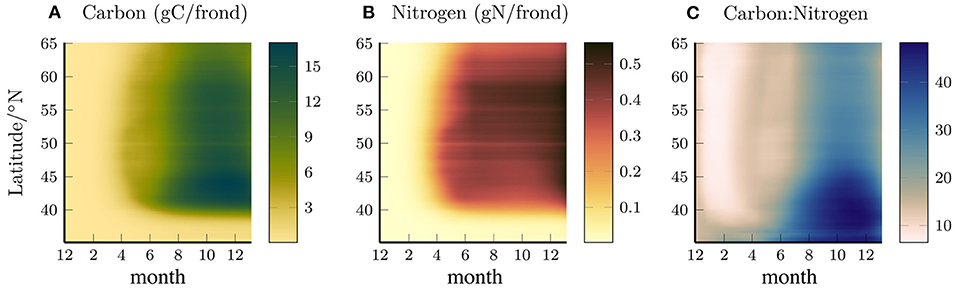# Sugar kelp (Saccharina latissima) individuals

We have implemented a model of sugar kelp growth within this spatially infinitesimal Lagrangian particles framework originally based on the model of Broch and Slagstad (2012) and updated by Broch et al. (2013), Fossberg et al. (2018), and Broch et al. (2019). This is the same model passively forced by Strong-Wright and Taylor (2022).

The model tracks three variables, the frond area, A (dm²), carbon reserve, C (gC / gSW), and nitrate reserve, N (gN / gSW). The growth depends on the nitrate (and optionally ammonia) availability in the water, the temperature, and light availability. The minimum required coupling is with nitrates so the model can be coupled with an NPZD model, but can optionally uptake ammonia, DIC (CO₂), oxygen, and release dissolved organic matter (from exudation) and large detritus.

Results could look something like this (from Strong-Wright and Taylor (2022)):## Model equations

As per Broch and Slagstad (2012) this model variables evolve as:

\begin{align} \frac{dA}{dt} & = \left(\mu - \nu\right)A, \\ \frac{dN}{dt} & = J - \mu(N + N_\text{struct}), \\ \frac{dC}{dt} & = P(1 - E) - R - \mu(C + C_\text{struct}). \end{align}

The apical frond loss given by:

$$$\nu = \frac{10^{-6}\exp(\varepsilon A)}{1 + 10^{-6}(\exp(\varepsilon A) - 1)}.$$$

The growth given by:

$$$\mu = f_\text{area}f_\text{seasonal}f_\text{temp}\min\left(\mu_c, \max(\mu_{NO_3}, \mu_{NH_4})\right),$$$

where:

\begin{align} f_\text{area} & = m_1\exp(-(A/A_0)^2) + m_2, \\ f_\text{seasonal} & = a_1(1 + \text{sgn}(\lambda(n))|\lambda(n)|^{1/2}) + a_2, \\ f_\text{temp} & = \left\{ \begin{array}{ll} 0.08T + 0.2 & -1.8\leq T < 10 \\ 1 & 10 \leq T \leq 15 \\ 19/4 - T/4 & 15 < T \leq 19 \\ 0 & T > 19 \end{array} \right\}, \end{align}

where $n$ is the day of the year, $\lambda$ is the normalised day length change, and $T$ is the temperature in degrees centigrade. The limiting rates ($\mu_c$, $\mu_{NO_3}$, $\mu_{NH_4}$) depend on the availability of carbon giving:

$$$\mu_c = 1 - \frac{C_\text{min}}{C},$$$

and on the available nitrogen which is either limited by the instantaneous uptake of ammonia, or the nitrogen reserve. To find these limits $J$, the nutrient uptake, must first be found (Fossberg et al., 2018). The uptake is calculated by first finding the $NO_3$ uptake rate:

$$$J_{NO_3} = J_{NO_3\text{, max}}f_\text{curr}\frac{N_\text{max} - N}{N_\text{max} - N_\text{min}}\frac{NO_3}{k_{NO_3} + NO_3},$$$

where $f_{curr} = c_1(1 - \exp(-u / c_2)) + c_3$ (Broch et al., 2019) where $u$ is the relative current speed. We then calculate the potential ammonia uptake:

$$$\tilde{J}_{NH_4} = J_{NH_4\text{, max}}f_\text{curr}\frac{NH_4}{k_{NH_4} + NH_4}.$$$

This results in a theoretical instantaneous area increase rate of:

$$$\mu_{NH_4} = \frac{1}{N_\text{min} + N_\text{struct}}\frac{\tilde{J}_{NH_4}}{k_A},$$$

or growth from reserves of:

$$$\mu_{NO_3} = 1 - \frac{N_\text{min}}{N}.$$$

The actual resulting ammonia uptake is then:

$$$J_{NH_4} = \min\left(\tilde{J}_{NH_4}, \mu k_A (N_\text{min} + N_\text{struct})\right),$$$

and the total uptake $J = \frac{J_{NH_4} + J_{NO_3}}{k_A}$.

The production of carbon from photosynthesis is given by:

$$$P = P_S\left[1 - \exp\left(\frac{\alpha PAR}{P_S}\right)\right]\exp\left(\frac{\beta PAR}{P_S}\right),$$$

where $PAR$ is the photosynthetically available radiation and

$$$P_S = \frac{\alpha I_\text{sat}}{\ln(1 + \alpha/\beta)}.$$$

$\alpha$ is a constant but $\beta$ depends on the maximum photosynthetic rate which is defined by both:

$$$P_\text{max} = \frac{\alpha I_\text{sat}}{\ln(1 + \alpha/\beta)}\left(\frac{\alpha}{\alpha + \beta}\right)\left(\frac{\beta}{\alpha + \beta}\right) ^ {\beta/\alpha},$$$

and

$$$P_\text{max} = \frac{P_1\exp\left(\frac{T_{AP}}{T_{P1}} - \frac{T_{AP}}{T}\right)}{1 + \exp\left(\frac{T_{APL}}{T} - \frac{T_{APL}}{T_{PL}}\right) + \exp\left(\frac{T_{APH}}{T} - \frac{T_{APH}}{T_{PH}}\right)},$$$

where $T$ is the temperature in kelvin and the $T_X$ are Arrhenius temperature constants. We solve these iteratively to find $\beta$.

The exudation fraction is given by:

$$$E = 1 - \exp\left(\gamma(C_\min - C)\right).$$$

As per Broch et al. (2013) $R$, the respiration rate, is given by:

$$$R = \left[R_A\left(\frac{\mu}{\mu_\text{max}} + \frac{J}{J_\text{max}}\right) + R_B\right]\exp\left(\frac{T_{ARR}}{T_1} - \frac{T_ARR}{T}\right).$$$

### Parameter variable names

SymbolVariable nameUnits
$A_0$growth_rate_adjustment1 / dm²
$\alpha$photosynthetic_efficiencygC / dm² / s / einstein
$C_\text{min}$minimum_carbon_reservegC / gSW
$C_\text{struct}$structural_carbongC / gSW
$\gamma$exudationgC / g
$\varepsilon$erosion1 / dm²
$I_\text{sat}$saturation_irradianceeinstein
$k_A$structural_dry_weight_per_areag / dm²
$k_dw$structural_dry_to_wet_weight-
$k_C$carbon_reserve_per_carbong / gC
$k_N$nitrogen_reserve_per_nitrogeng / gN
$N_\text{min}$minimum_nitrogen_reservegN / gSW
$N_\text{max}$maximum_nitrogen_reservegN / gSW
$m_2$growth_adjustment_2-
$m_1$growth_adjustment_1-
$\mu_\text{max}$maximum_specific_growth_rate1 / s
$N_\text{struct}$structural_nitrogengN / gSW
$P_1$photosynthesis_at_ref_temp_1gC / dm² / s
$P_2$photosynthesis_at_ref_temp_2gC / dm² / s
$T_{P1}$photosynthesis_ref_temp_1°K
$T_{P2}$photosynthesis_ref_temp_2°K
$a_1$photoperiod_1-
$a_2$photoperiod_2-
$R_1$respiration_at_ref_temp_1gC / dm² / s
$R_2$respiration_at_ref_temp_2gC / dm² / s
$T_{R1}$respiration_ref_temp_1°K
$T_{R2}$respiration_ref_temp_2°K
$T_{AP}$photosynthesis_arrhenius_temp°K
$T_{PL}$photosynthesis_low_temp°K
$T_{PH}$photosynthesis_high_temp°K
$T_{APL}$photosynthesis_high_arrhenius_temp°K
$T_{APH}$photosynthesis_low_arrhenius_temp°K
$T_{ARR}$respiration_arrhenius_temp°K
$u_{0p65}$current_speed_for_0p65_uptakem / s
$k_{NO_3}$nitrate_half_saturationmmol N / m³
$k_{NH_4}$ammonia_half_saturationmmol N / m³
$J_{NO_3\text{, max}}$maximum_nitrate_uptakegN / dm² / s
$J_{NH_4\text{, max}}$maximum_ammonia_uptakegN / dm² / s
$c_1$current_1-
$c_2$current_21 / m / s
$c_3$current_3-
$R_A$respiration_reference_AgC / dm² / s
$R_B$respiration_reference_BgC / dm² / s
-exudation_redfield_ratiommol C / mmol N

All default parameter values are given in Parameters.

## Model conservations

Total nitrogen (and carbon where appropriate) are conserved between the individuals and biogeochemistry. The total nitrogen in each individual is:

$$$N_\text{total} = Ak_A(N + N_\text{struct}),$$$

and carbon:

$$$C_\text{total} = Ak_A(C + C_\text{struct}).$$$